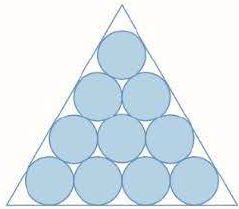Chapter 11, Problem 15P

Chapter
Section
Textbook Problem

# Suppose that circles of equal diameter are packed tightly in n rows inside an equilateral triangle. (The figure illustrates the case n = 4.) If A is the area of the triangle and An is the total area occupied by the n rows of circles, show that lim n → ∞ A n A = π 2 3 FIGURE FOR PROBLEM 15To determine

To show: The limnAnA=π23 .

Explanation

Given:

The circles of the equal diameter are packed tightly in n rows inside an equilateral tri-angle.

Calculation:

Consider L is the length of the equilateral tri-angle, then the area is shown below,

A=12L34L243A=L2

There are n rows of the circle and r be the radius of one of the circle.

L=3r+r+(n2)(2r)+r+3r=r(2n2+23)r=L(2n2+23)

Therefore the number of circle is shown below,

1+2+3+n=n(n+1)2

Hence the total area of  the circle is An .

An=n(n+1)2πr2=n(n+1)2π(L(2n2+23))2=n(n+1)2πL24(n31)2=n(n+1)2π4A34<

### Still sussing out bartleby?

Check out a sample textbook solution.

See a sample solution

#### The Solution to Your Study Problems

Bartleby provides explanations to thousands of textbook problems written by our experts, many with advanced degrees!

Get Started

#### Simplify the expressions in Exercises 97106. (xy)1/3(yx)2/3

Finite Mathematics and Applied Calculus (MindTap Course List)

#### Evaluate the integral. 45. 0/61+cos2xdx

Single Variable Calculus: Early Transcendentals

#### 0 1 ∞

Study Guide for Stewart's Multivariable Calculus, 8th